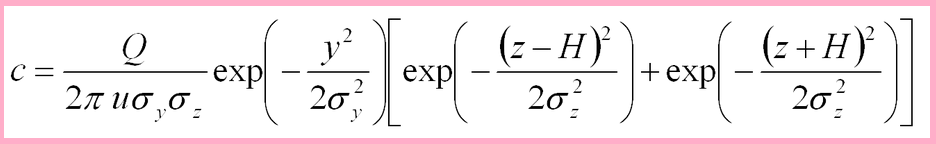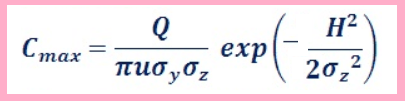#Gaussian Equation Calculator

This online gaussian plume dispersion equations and gaussian Cmax will help you to design the stacks & environmental impact analysis.

π = 3.141592653589793238

Step by Step calculation## Gaussian Plume Dispersion Equations Formulas

This below equation assumes that Dispersion will continue vertically even below the ground level.

The truth is that vertical spreading terminates at ground level.

This method is equivalent to assuming that a mirror iimage plume exists below the ground.

The added new concentration due to the image plume uses z+H instead of z-H.## Gaussian Max Ground Level Emission Concentration Formulas

The ground-level concentrations directly downwind are of interest, since pollution concentration will be highest along that axis.

Using the below equation , the effect of variations in the key parameters (atmospheric stability, wind speed, ambient temperature, stack height, gas exit velocity, and gas exit temperature) on the ground concentrations are calculated.where,

C is maximum ground level concentration of emission at point(x,y,z) in μg/m3

Q is emission rate μg/s.

σy is horizontal dispersion of emission in m.

σz is vertical dispersion of emission in m.

π = 3.141592653589793238

U is average wind speed at stack height in m/s.

H is effective stack height in m.

H = ∆h + h

∆h is plume rise in m.

h is physical stack height in m.

σz is H ÷ √2 for neutral atmospheric conditions.